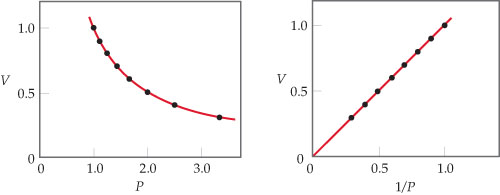# Problem: Boyles Law: For a fixed quantity of gas at constant temperature, the volume of the gas is inversely proportional to its pressure.What would a plot of P versus 1/V look like for a fixed quantity of gas at a fixed temperature?

###### FREE Expert Solution

Recall that the ideal gas law is:

$\overline{){\mathbf{PV}}{\mathbf{=}}{\mathbf{nRT}}}$

The pressure and volume of a gas are related to the number of moles, gas constant and temperatureThe value nRT is constant

$\overline{){\mathbf{PV}}{\mathbf{=}}{\mathbf{constant}}}$

100% (181 ratings)###### Problem DetailsBoyles Law: For a fixed quantity of gas at constant temperature, the volume of the gas is inversely proportional to its pressure.

What would a plot of P versus 1/V look like for a fixed quantity of gas at a fixed temperature?

Frequently Asked Questions

What scientific concept do you need to know in order to solve this problem?

Our tutors have indicated that to solve this problem you will need to apply the Chemistry Gas Laws concept. You can view video lessons to learn Chemistry Gas Laws. Or if you need more Chemistry Gas Laws practice, you can also practice Chemistry Gas Laws practice problems.